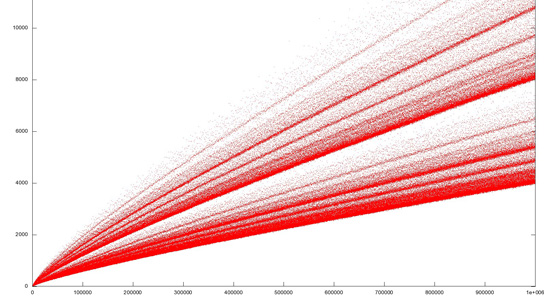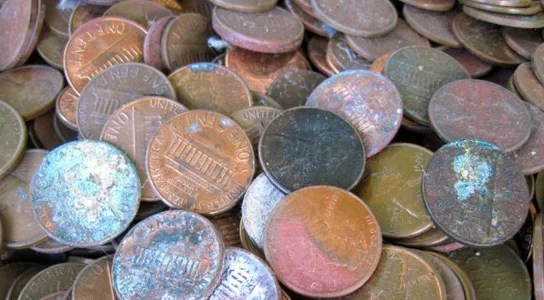## New Mathematical Proof of the ABC Conjecture

A new claim could imply that a proof of one of the most important conjectures in number theory has been solved, which would be an astounding achievement. Mathematician Shinichi Mochizuki of Kyoto University in Japan has released a 500-page proof of the abc conjecture that proposes a relationship between whole numbers (related to the Diophantine equations).

## Century-Old Goldbach Weak Conjecture Closer to Being SolvedThe weak Golbach conjecture states that you can break up any odd number into the sum of, at most, three prime numbers. Prime numbers cannot be evenly divided by any other number than themselves or 1.

## Probabilities of Collecting All Pennies from 1959 to 1997 are Easily FeasibleMany coin collectors start their numismatic collections with pennies, and trying to get pennies from every year within a given range. This process, while seemingly daunting, is straightforward and will take about 684 pennies to find all of the pennies from 1959 to 1997, since they are still in circulation.

## The Algorithmic Approach to the Mathematics of CrammingStudents tend to take far from optimal ways to assimilate information, especially when it comes to exams. Scientists Tim Novikoff, Jon Kleinbert, and Steve Strogatz decided to take a mathematical approach to the way that students learn, in order to find the most effective way to succeed.

## The Fractal Dimension of the US ZIP Code System: 1.78!While most people think that ZIP codes were originally created randomly, there is actually an order to the US mail system. Since it turns out that it has a branching structure, it implies that it has a fractal dimension. Samuel Arbesman of Wired’s Social Dimension used the ZIPScribbles images created by Robert Kosara to calculate the fractal dimension of the US ZIP code system.

## Mathematician Claims Breakthrough in the Sudoku ProblemWhile you might just need a pencil and your brain to fill in this week’s Sudoku puzzle, an Irish mathematician used millions of hours of supercomputing time in order to solve an important open problem in the mathematics of Sudoku; the game that was initially popularized in Japan and involves filling up a 9×9 grid with the numbers 1 to 9 according to certain rules.